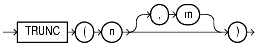## TRUNC (number)

#### Syntax

trunc_number::=Text description of trunc_number

#### Purpose

The `TRUNC` (number) function returns `n` truncated to `m` decimal places. If `m` is omitted, then `n` is truncated to 0 places. `m` can be negative to truncate (make zero) `m` digits left of the decimal point.

#### Examples

The following example truncate numbers:

```SELECT TRUNC(15.79,1) "Truncate" FROM DUAL;

Truncate
----------
15.7
```
```SELECT TRUNC(15.79,-1) "Truncate" FROM DUAL;

Truncate
----------
10
```